Worksheet Solutions: Triangles

# Worksheet Solutions: Triangles - Mathematics (Maths) Class 9

### Multiple Choice Questions

Q1: If AD = BC and ∠ BAD = ∠ ABC, then ∠ ACB is equal to
(a) ∠ABD
(c) ∠BAC
(d) ∠BDA
Ans: (d)
In △ABC and △ABD
∠ BAD = ∠ ABC    (Given)
AB = AB   (Common side)
∴ △ABC ≅ △ABD
By CPCT theorem, ∠ACB=∠BDA ( By SAS Congruency)

Q2: If O is a midpoint of AB and ∠BQO = ∠APO, then ∠OAP is equal to
(a) ∠QPA
(b) ∠OQB
(c) ∠QBO
(d) ∠BOQ
Ans:
(c)
In △AOP and △BOQ
AO = BO….. (O is the midpoint of AB)
∠APO = ∠BQO (Given)
∠AOP = ∠BOQ (Vertically opposite Angles)
∴△AOP ≅ △BOQ (By AAS Congruency)
By CPCT ∠OAP = ∠QBO

Q3: If △ABC is an isosceles triangle, ∠ B = 650, find ∠ A.
(a) 60º
(b) 70º
(c) 50º
(d) none of these
Ans:
(c)
Since △ABC is on an isosceles triangle
∴ ∠B = ∠C
∴ ∠B = 65º
∴ ∠C = 65º
∴ ∠A+∠B +∠C = 180º
∴ ∠A + 130º = 180º
∴ ∠A = 180º − 130º
∴∠A = 50º

Q4: An angle is 140 more than its complement. Find its measure.
(a) 42
(b) 32
(c) 52
(d) 62
Ans:
(c)
Two angles whose sum equals 90 degrees are called complementary angles.
let first angle = x
it’s Complement = 90º − x
According to the question,
x = 14º + 90º − x
x = 104 − x
⇒ 2x = 104º
⇒ x = 1042
∴ x = 52º

Q5: If ABCD is a quadrilateral where AD = CB, AB = CD, and ∠ D = ∠ B, then ∠CAB is equal to
(a) ∠ACD
(c) ∠ACD
Ans:
(c)
In △ABC and △CDA
AB = CD    (Given)
∠B = ∠D    (Given)
∴△ABC ≅ △CDA       (By SAS Congruency)
By CPCT theorem
∠CAB = ∠ACD

Q6: If AB ⊥BC and ∠A =∠C, then the correct statement will
(a) AB ≠ AC
(b) AB = BC
(d) AB = AC
Ans:
(b)
In △ABC, ∠A = ∠C
Opposite sides to equal angles are also equal
AB = BC

Q7: If AB = AC and ∠ ACD = 1200, find ∠A.
(a) 500
(b) 600
(c) 700
(d) none of these
Ans:
(b)
Since AB = AC
⇒∠ABC = ∠ACB = x (say)
Let ∠BAC = y
We know,
Exterior angles = sum of interior opposite angles
120º = ∠ABC + ∠BAC
120º = x + y−−−(1)
Again, ∠ACB + ∠ACD = 180º
x+120º = 180º
∴ x = 60º
From(1),
60º + y = 120º
⇒ y = 60º
⇒ ∠A = 60º

Q1: AD and BC are equal perpendiculars to a line segment AB. Show that CD bisects AB.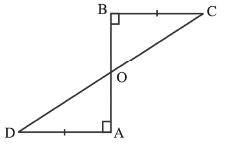Ans: In ΔAOD and ΔBOC,
BC || AD (alternate angles are equal)
∠ODA = ∠OCB.
So, ΔAOD ≅ ΔBOC (by the ASA congruence rule) OD = OC (CPCT)
and these form a pair of alternate angles for line segments AD and BC.
Therefore, CD bisects AB.

Q2: AB is a line segment and P is its mid-point. D and E are points on the same side of AB such that ∠BAD = ∠ABE and ∠EPA = ∠DPB. Show that
(i) ΔDAP ≌ ΔEBP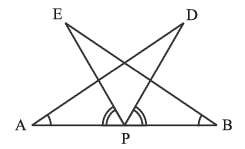Ans. (i) ΔIn DAP and ΔEBP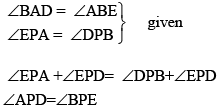P is its mid-point of AB
∴ AP = PB
So, ΔDAP ≌ ΔEBP(by the ASA congruence rule)

Q3: In an isosceles triangle ABC with AB = AC, D and E are points on BC such that BE = CD. Show that AD = AE.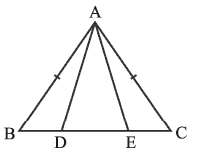Ans:
In ΔABD and ΔACE,
AB = AC (Given) ..(1)
∠B = ∠C (Angles opposite to equal sides) ..(2)
Also, BE = CD..(3)
So, BE – DE = CD – DE
That is, BD = CE (3)
So, ΔABD ≌ ΔACE
(Using (1), (2), (3) and SAS rule).
This gives AD = AE (CPCT)

Q4: In Figure OA = OB and OD = OC.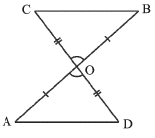Show that
(i) ΔAOD ≅ ΔBOC
Ans:
(i) In ΔAOD and ΔBOC,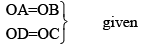∠AOD = ∠BOC.(pair of vertically opposite angles)
So, ΔAOD ≅ ΔBOC(by the SAS congruence rule)
and these form a pair of alternate angles for line segments AD and BC.

Q5: In Fig, AC = AE, AB = AD and ∠BAD = ∠EAC. Show that BC = DE.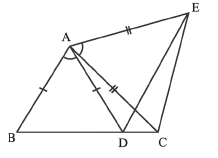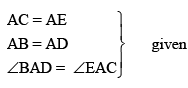∠BAC = ∠DAE
So, ΔABC ≌ ΔADE(by the SAS congruence rule)
BC = DE (CPCT)

Q6: In ΔABC, the bisector AD of ∠A is perpendicular to side BC. Show that AB = AC and ΔABC is isosceles.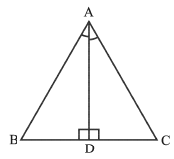Ans:
So, ΔABD ≌ ΔADC (by the SAS congruence rule)
AB = AC(CPCT)
∴ ΔABC is isosceles

Q7: ABC is a triangle in which altitudes BE and CF to sides AC and AB are equal. Show that
(i) ΔABE ≌ ΔACF
(ii) AB = AC, i.e., ABC is an isosceles triangle.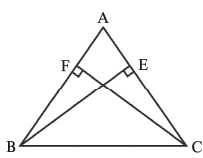Ans: In ΔABE and ΔACF,
BE = FC (Given) ..(1)
∠A = ∠A (Common Angle) ..(2)
Also, ∠ABE = ∠ACF  ....(3)
So, ΔABE ≌ ΔACF(Using (1), (2), (3) and ASA rule).
This gives AB = AC (CPCT)
∴ ABC is an isosceles triangle

The document Worksheet Solutions: Triangles | Mathematics (Maths) Class 9 is a part of the Class 9 Course Mathematics (Maths) Class 9.
All you need of Class 9 at this link: Class 9

## Mathematics (Maths) Class 9

42 videos|378 docs|65 tests

## FAQs on Worksheet Solutions: Triangles - Mathematics (Maths) Class 9

 1. What are the different types of triangles?Ans. There are three main types of triangles: equilateral triangles, isosceles triangles, and scalene triangles. An equilateral triangle has three equal sides and three equal angles of 60 degrees each. An isosceles triangle has two equal sides and two equal angles. A scalene triangle has three unequal sides and three unequal angles.
 2. How do you find the area of a triangle?Ans. To find the area of a triangle, you can use the formula A = (base * height) / 2. The base of a triangle is one side of the triangle, and the height is the perpendicular distance from the base to the opposite vertex.
 3. What is the Pythagorean theorem?Ans. The Pythagorean theorem states that in a right-angled triangle, the square of the length of the hypotenuse (the side opposite the right angle) is equal to the sum of the squares of the lengths of the other two sides. It is represented by the equation a^2 + b^2 = c^2, where 'a' and 'b' are the lengths of the two legs and 'c' is the length of the hypotenuse.
 4. How can you determine if three given side lengths form a triangle?Ans. To determine if three given side lengths form a triangle, you can use the triangle inequality theorem. According to this theorem, the sum of the lengths of any two sides of a triangle must be greater than the length of the third side. If this condition is satisfied for all three combinations of sides, then the given lengths can form a triangle.
 5. What are the properties of similar triangles?Ans. Similar triangles have the following properties: 1. Corresponding angles are equal: The angles of one triangle are equal to the corresponding angles of another triangle. 2. Corresponding sides are proportional: The ratio of the lengths of corresponding sides in two similar triangles is equal. This ratio is known as the scale factor. 3. The corresponding altitudes, medians, and angle bisectors are proportional. 4. The areas of similar triangles are proportional to the square of the scale factor.

## Mathematics (Maths) Class 9

42 videos|378 docs|65 testsExplore Courses for Class 9 examSignup to see your scores go up within 7 days! Learn & Practice with 1000+ FREE Notes, Videos & Tests.
10M+ students study on EduRev
Track your progress, build streaks, highlight & save important lessons and more!
Related Searches

,

,

,

,

,

,

,

,

,

,

,

,

,

,

,

,

,

,

,

,

,

;